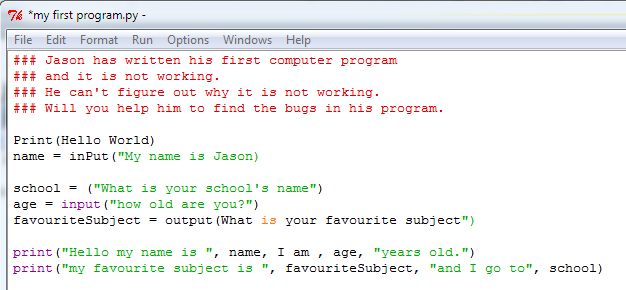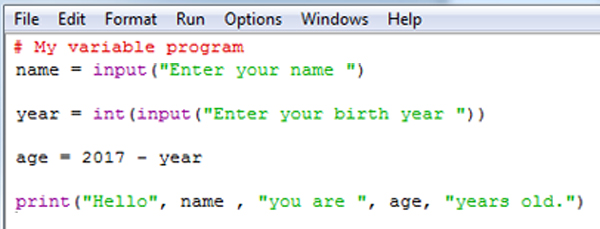# Programming with Python L4

## LESSON 4

### Lesson Objectives

• Learning how to deal with user input and learn about different user input types

### Success Criteria

• I understand how to use a variable
• I know how to capture user input
• I can use basic data types, string, integer, float and Boolean.

• String
• Integer
• Boolean
• Floats

• input()
• int()
• print()
• float()

### Starter

Help Jason to get his program working.Open your Python program from start button > all apps > Python 3.1 > select IDLE (Python GUI) in the script mode rewrite the code below with the correct syntax. Once you have make it working write the final code in your books with annotating what corrections you have made.Remember correct input syntax should be input() and print syntax should be print() and  string text should be inside the speech marks.

### Task 2 what is input?

In your previous lesson, you have learnt how to store variables.

For example in the following code user enter their name date of birth year:Then What happens?

Modify this above code that your new program stores different variables and it asks for different input.

• Ask user their favourite film
• Ask user if they support Arsenal or any other football team
• Ask if they have any siblings

Save your program and then Run it (F5)

And your code should printout all the responses like in following example.### Data Types

While coding, it is important to use the correct syntax for a correct data type.

For example, you wouldn’t try to save your name in a number data type. It would not be compatible. And you will either have an error or unwanted result from your code output.

Here are some of the data type available to us:

• Integer
• String
• Float
• Boolean

A string is a data type generally used for a sequence of characters. Which we usually refer them as text. An example of a string data type is shown below.

“Hello World”

“Welcome to the Planet Earth”

We use string data always with double speech marks.

to input string into your program, you should use the input command.

input()

Explain in your book what is String data type (use green pen)

An integer is a datatype generally used for numbers. A value stored in the datatype is usually positive or negative.

Example integer inputs would be:

• 6
• 101
• -5
• 9999

To include input from an integer, you would use the following code:

int(input( ))

Explain in your book what is Integer data type (use green pen)

The best way to remember float is that it is used so that we can get a more accurate result. We tend to use float when working with decimal numbers.

Example floats inputs would be:

• 2.5
• 12.80
• -5.15
• 355.75

To include input from an float, you would use the following code:

float(input( ))

Explain in your book what is Float data type (use green pen)

When carrying out tests in life, things are usually right or wrong. There is no blurred lines in between. You either saved your work or you didn’t.

Booleans are a data type which test two outcomes. True or False.

Remember from your maths lessons what does it mean these symbols:

• >
• <
• >=
• <=
• in python this == symbol means equal. This = does not mean equal this is something you assigned data.
• in python this != means not equal.

Write in your books below examples and outcomes. First one is done for you.

1. 55 < 99 this will be TRUE because 55 is smaller than 99
2. 5 > 10
3. 21 >= 21
4. 10 != 22
5. 43 < = 45

There are various ways of including Boolean data in your code very simple on is using if. Below is an example of using Boolean condition with if:
What do you think will be the outcome of these codes.
if 9 > 11:
print(“Correct”)
else:
print(“Incorrect”)

or

day = input(“What day is today?”)
if day == “Monday” :
print(“Week is just begun”)
else:
print(“Weekend is close, just keep it going “)

### Remember!

Always code in SCRIPT mode
(i.e. go to FILE, NEW WINDOW once you have finish coding save your program and to run it press F5)

Write a program asking user to enter different data types and displays the outcome.

• Integer use int(input())
• String use input()
• Float use float(input())
• Boolean and test the way shown above using if structure

you can ask user to enter two integer numbers and

num1 =int(input(“Enter a number “)

divNum = float(num1/num2)

Extension

See if you can combine multiple data types on one line. You should create a story with the use of inputs. For example, name, age, location, monstername. Once all the inputs have been typed the story is shown including the inputs.